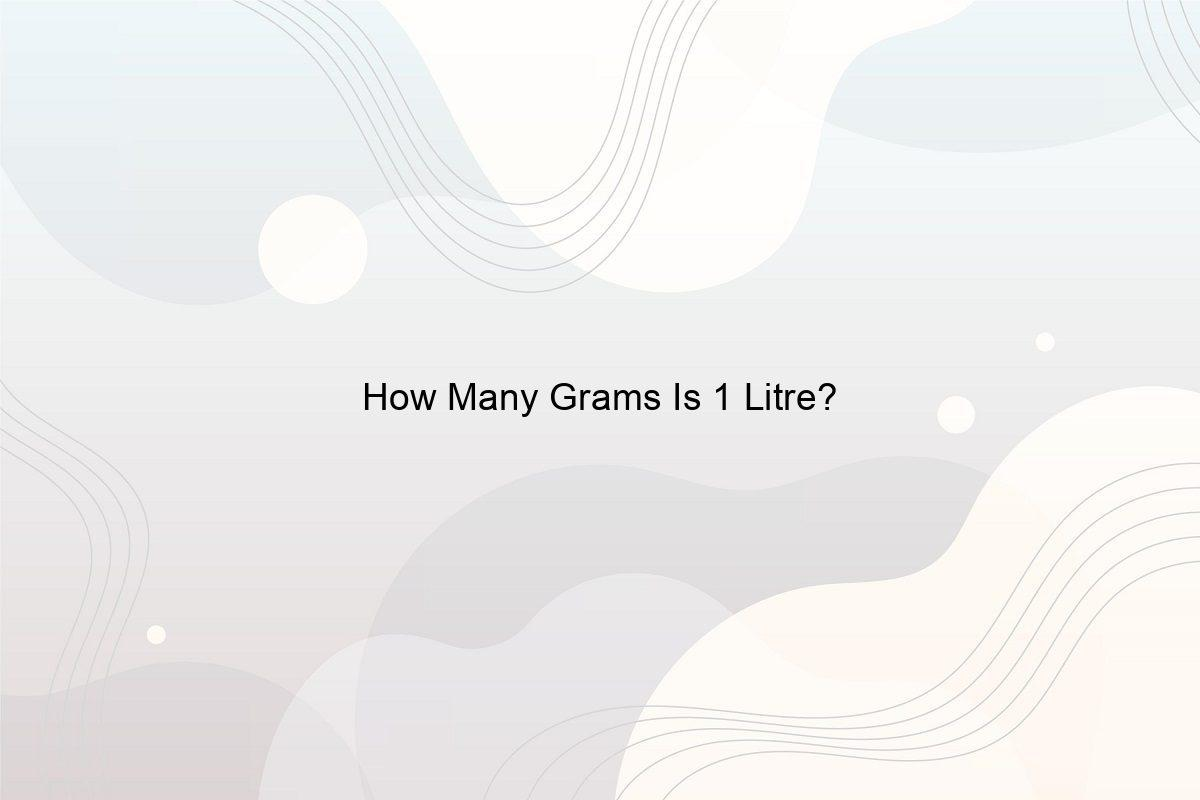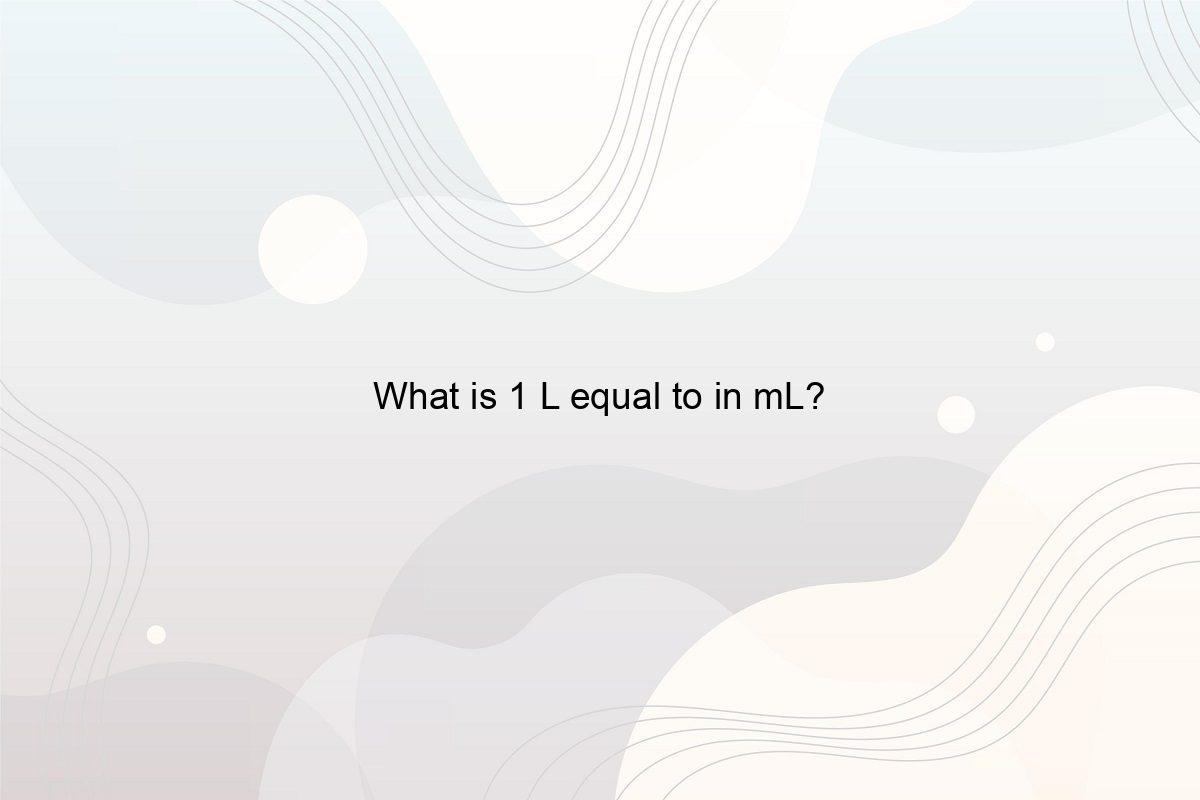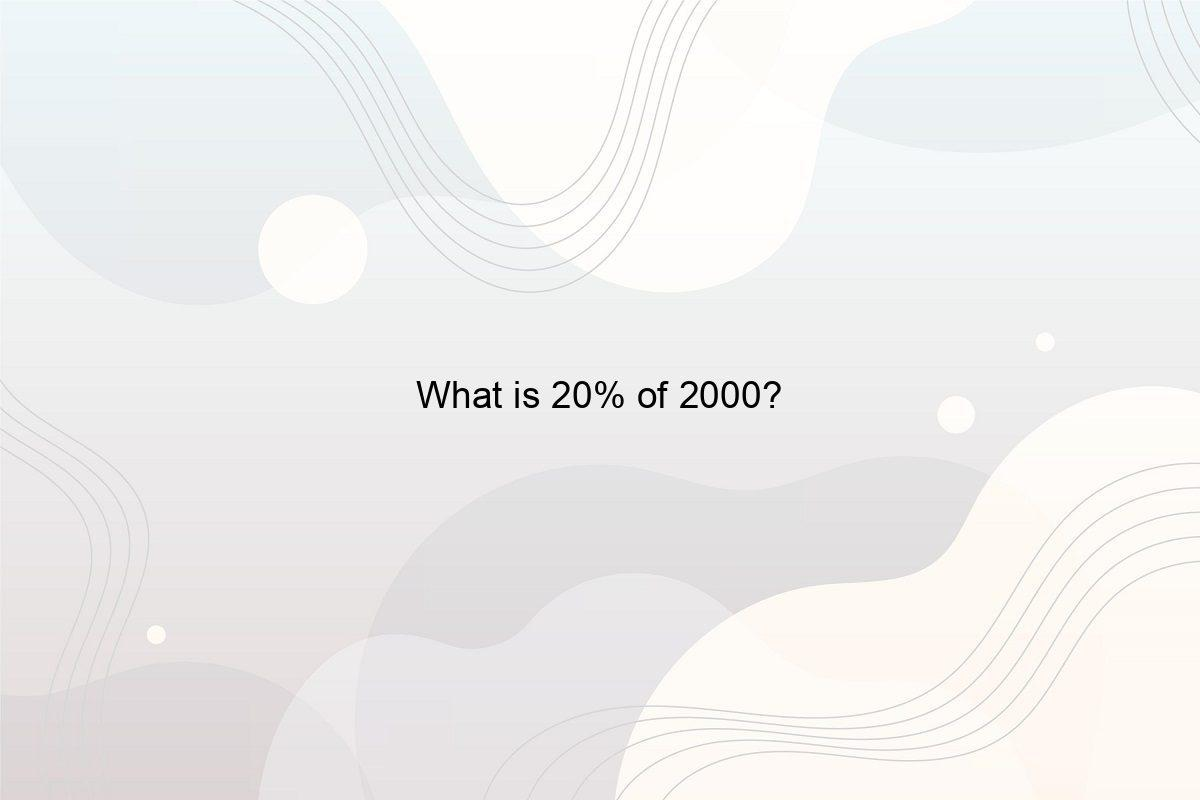﻿ What is 1/4 plus 1/4? - Speeli

# What is 1/4 plus 1/4?

What is one-fourth plus one-fourth? Fraction calculator: 1/4+1/4
1. ## What figure will you get when you add 1/4 to 1/4?

You can work with these numbers the same way you do with whole numbers. Even though fractions depict less than one number, they can still be added, subtracted, multiplied, and divided. The primary thing that makes the operations with fractions easier is ensuring whether the fractions have the same denominator or not.

So, when you add both the numbers, you will get:

1/4 + 1/4 = 2/4 = 1/2 (As when you divide 2 with 4, you will get 1/2 as the answer)
As the denominator was the same in both the above fractions, the calculation could be made effortlessly. So, jotting down in words, one fourth plus one fourth is half.

2. ## What are the examples of some fractions equivalent to half and one fourth?

Fractions equivalent to 1/2 are 1/2, 2/4, 3/6, 8/16, 15/30, and so on. Whereas fractions equivalent to 1/4 are 1/4, 2/8, 3/12, 5/20, 10/40, and the series continues.

##### Related Posts## How Many Grams Is 1 Litre?

Are Grams and Litres the same? How many Litres is 1 kg? How many Grams is 1 litre? How to Convert 1 litre to Grams?## How much is 2 quarts in ounces?

How much is 2 quarts of water in cups? Multiplying the value of quart by 32 will give the result in the number of ounces it has. Hence, the answer here is 64.